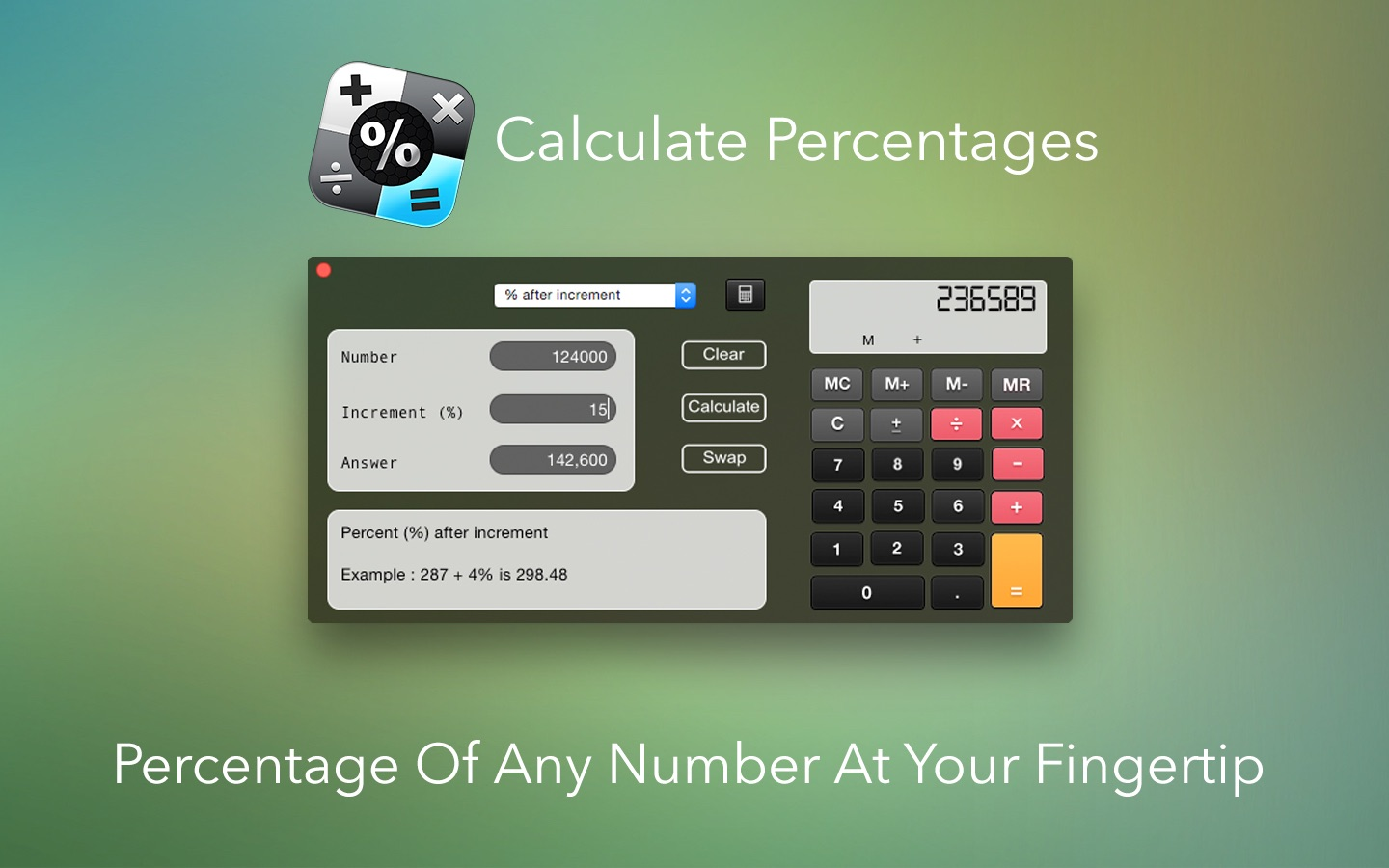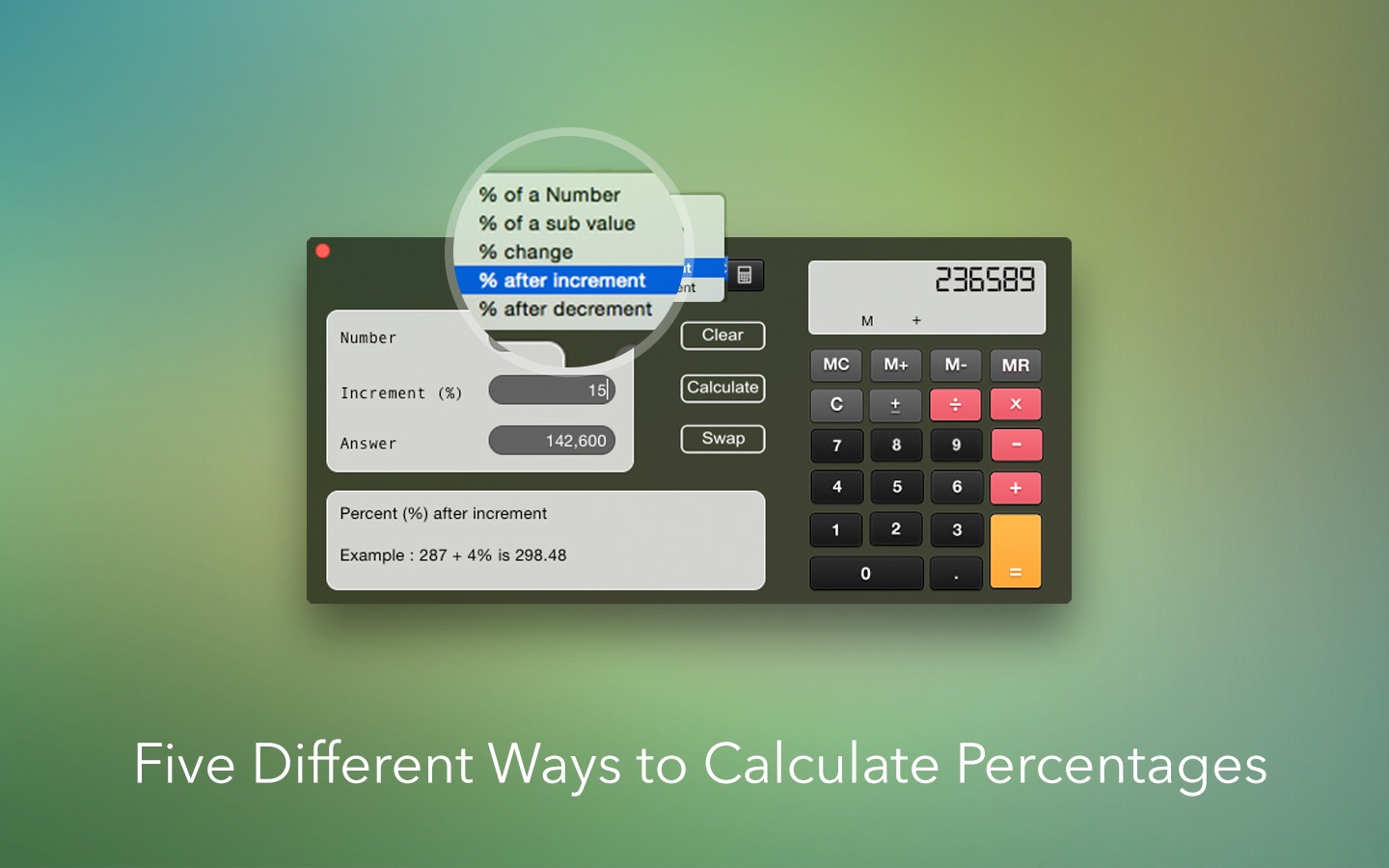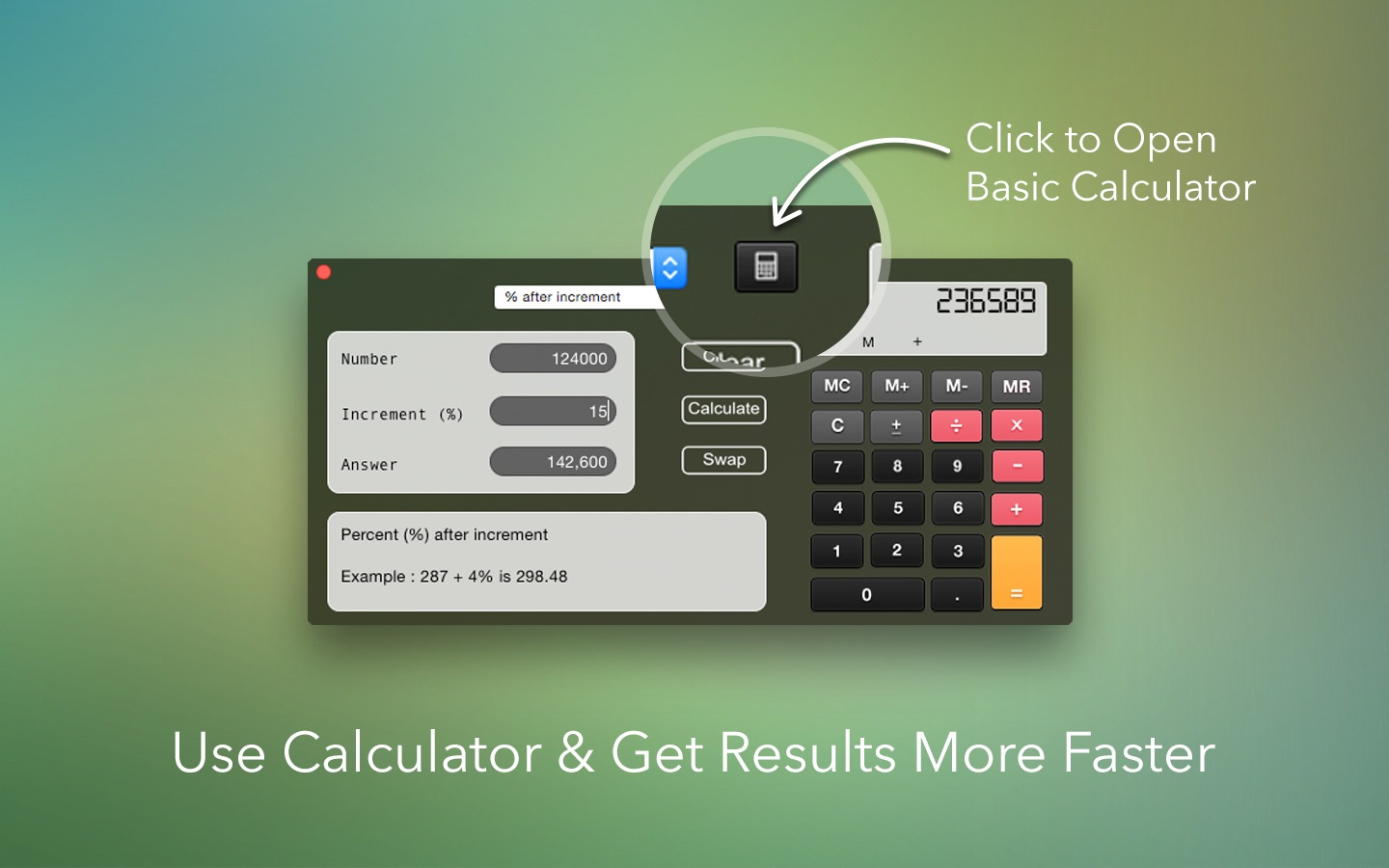iLifeTouch | Making life easier one day at a time...
•••## Calculate Percentages

Calculate Percentages isn't just an app that calculates five different types of percentages but it also is a tutorial with visible examples of exactly what it is you are calculating a percentage of.

This extra level of detail makes Calculate Percentages so extremely user friendly. A tutorial app with examples to compare your calculations against is more valuable than an app that simply calculates numbers.

Calculate Percentages also has a built in Calculator that allows all types of calculations.

This app is also available on the go for iPhone and iPad!!!

Productivity